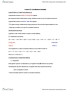# CHEM102 Lecture Notes - Lecture 5: Arrhenius Equation, Collision Frequency, Collision Theory

47 views4 pages
School
Department
Course
Professorcccchrissyy and 37705 others unlocked32
Verified Note
32 documents

## Document Summary

There is an energy barrier that the molecules must overcome. Rate of reaction is sensitive to change in temperature. In most reactions: the change in rate constant, k to the temperature (k) is exponential, for many reactions, an increase of 10oc in temperature doubles the reaction rate. Put forward by arrhenius in 1889, it is in the form of. Equation links the rate constant k with activation energy, ea and temperature, t k = ae-ea/rt. A = arrhenius constant and represents collision frequency factor. (how easy do the molecules collide with each other. ) Ea = activation energy of the reaction / j mol-1 or kj mol-1 e = base of natural logarithm and is equal to 2. 718. R = gas constant and is equal to 8. 314 j k-1 mol-1. The minimum energy the molecules must possess for a chemical reaction to take place is called the activation energy.